# Introduction to Arrays Useful Array Operations o Finding

• Slides: 55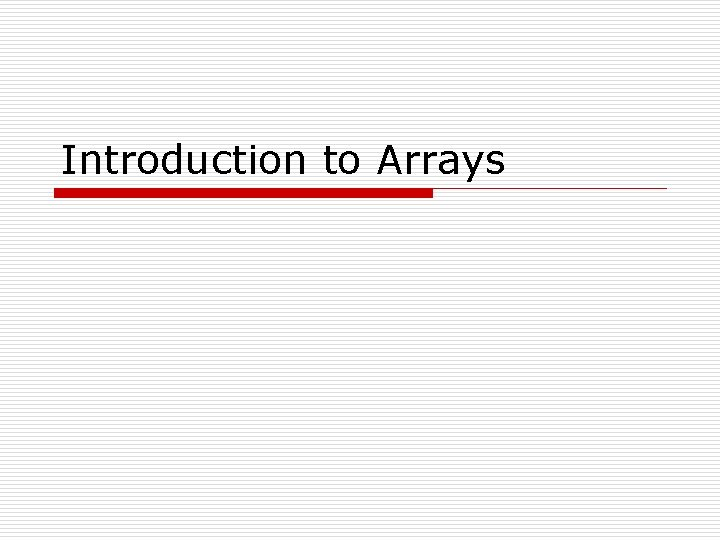Introduction to ArraysUseful Array Operations o Finding the Highest Value int [] numbers = new int; int highest = numbers; for (int i = 1; i < numbers. length; i++) { if (numbers[i] > highest) highest = numbers[i]; } o Finding the Lowest Value int lowest = numbers; for (int i = 1; i < numbers. length; i++) { if (numbers[i] < lowest) lowest = numbers[i]; }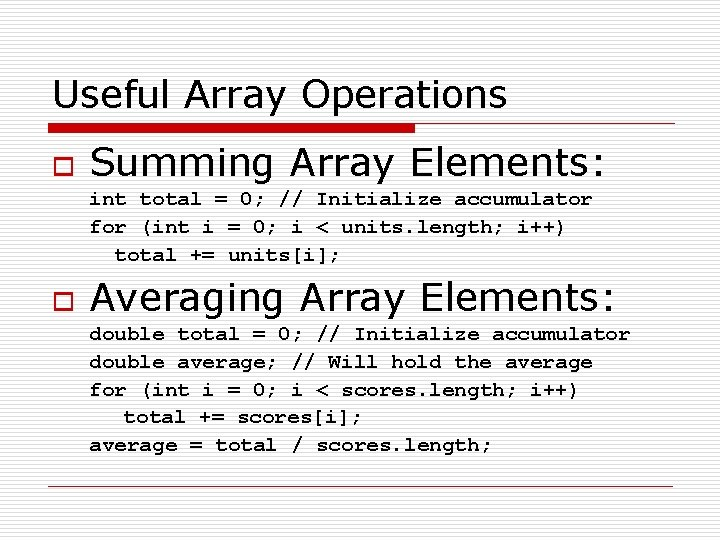Useful Array Operations o Summing Array Elements: int total = 0; // Initialize accumulator for (int i = 0; i < units. length; i++) total += units[i]; o Averaging Array Elements: double total = 0; // Initialize accumulator double average; // Will hold the average for (int i = 0; i < scores. length; i++) total += scores[i]; average = total / scores. length;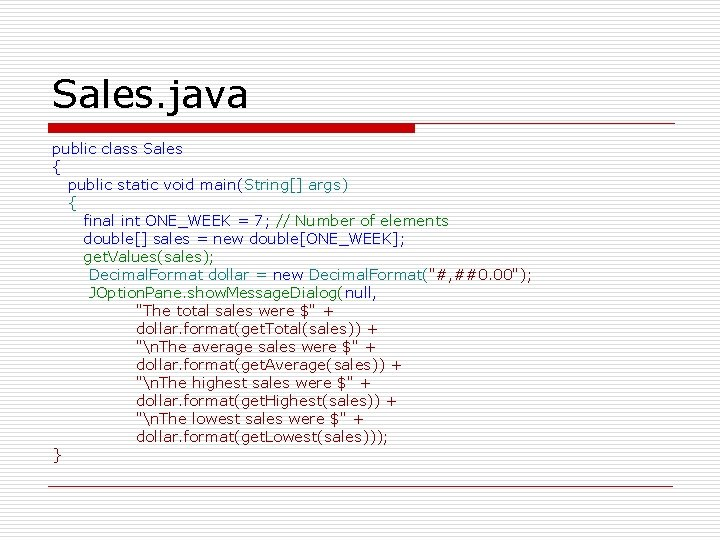Sales. java public class Sales { public static void main(String[] args) { final int ONE_WEEK = 7; // Number of elements double[] sales = new double[ONE_WEEK]; get. Values(sales); Decimal. Format dollar = new Decimal. Format("#, ##0. 00"); JOption. Pane. show. Message. Dialog(null, "The total sales were \$" + dollar. format(get. Total(sales)) + "n. The average sales were \$" + dollar. format(get. Average(sales)) + "n. The highest sales were \$" + dollar. format(get. Highest(sales)) + "n. The lowest sales were \$" + dollar. format(get. Lowest(sales))); }public static double get. Total(double[] sales ) { double total = 0. 0; // Accumulator } for (int index = 0; index < sales. length; index++) total += sales[index]; return total; public static double get. Average(double[] sales ) { return get. Total() / sales. length; }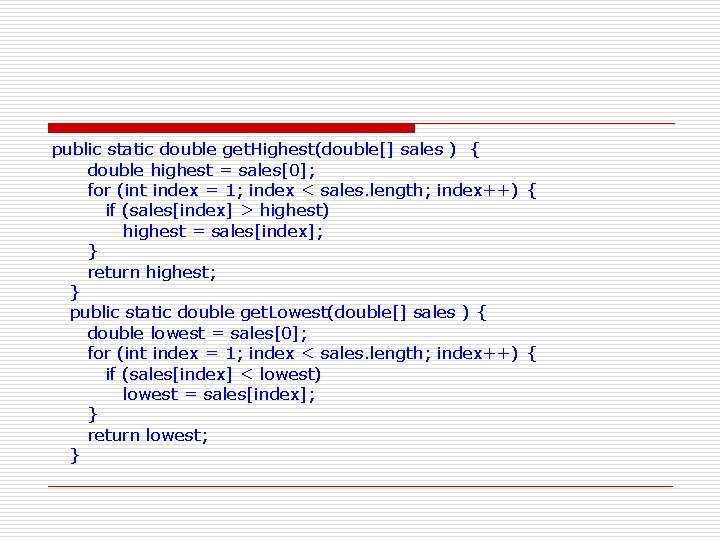public static double get. Highest(double[] sales ) { double highest = sales; for (int index = 1; index < sales. length; index++) { if (sales[index] > highest) highest = sales[index]; } return highest; } public static double get. Lowest(double[] sales ) { double lowest = sales; for (int index = 1; index < sales. length; index++) { if (sales[index] < lowest) lowest = sales[index]; } return lowest; }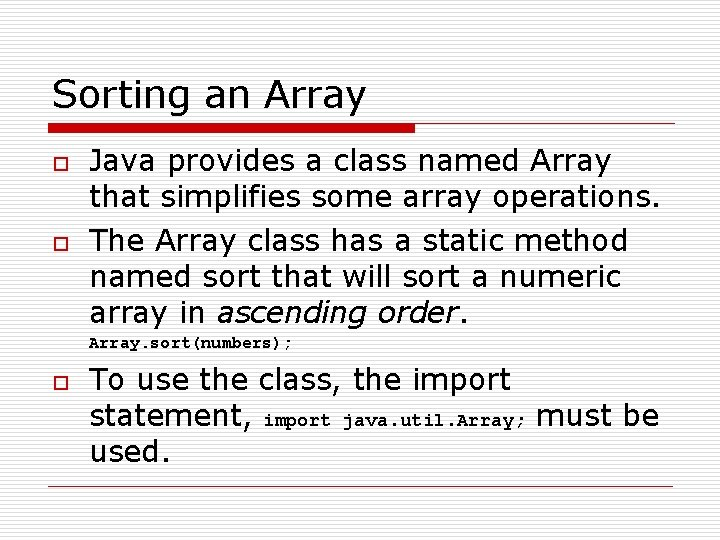Sorting an Array o o Java provides a class named Array that simplifies some array operations. The Array class has a static method named sort that will sort a numeric array in ascending order. Array. sort(numbers); o To use the class, the import statement, import java. util. Array; must be used.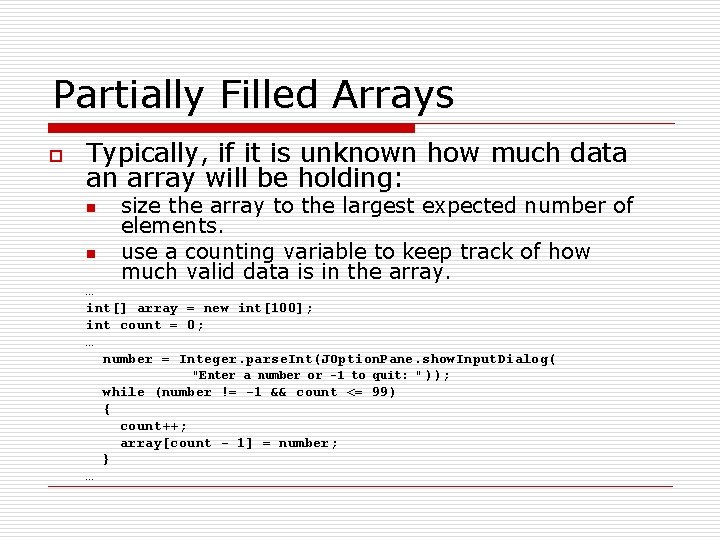Partially Filled Arrays o Typically, if it is unknown how much data an array will be holding: n n size the array to the largest expected number of elements. use a counting variable to keep track of how much valid data is in the array. … int[] array = new int; int count = 0; … number = Integer. parse. Int(JOption. Pane. show. Input. Dialog( "Enter a number or -1 to quit: " )); while (number != -1 && count <= 99) { count++; array[count - 1] = number; } …Returning an Array Reference o o A method can return a reference to an array. The return type of the method must be declared as an array of the right type. public static double[] get. Array() { double[] array = { 1. 2, 2. 3, 4. 5, 6. 7, 8. 9 }; return array; } o The get. Array method is a public static method that returns an array of doubles.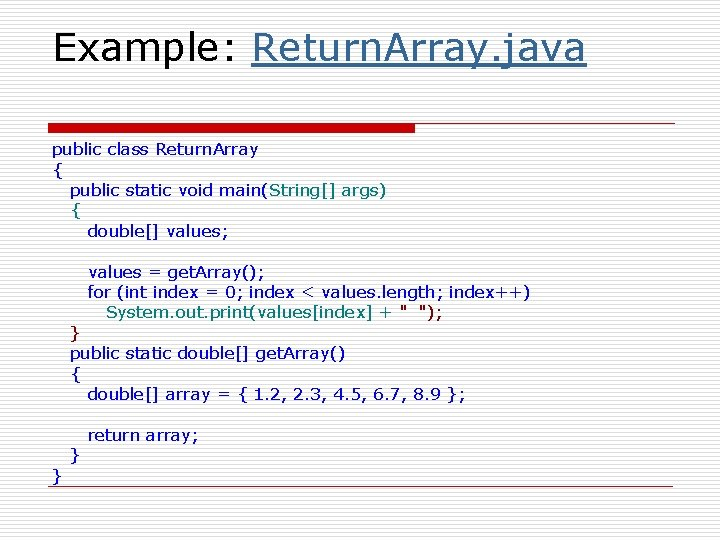Example: Return. Array. java public class Return. Array { public static void main(String[] args) { double[] values; values = get. Array(); for (int index = 0; index < values. length; index++) System. out. print(values[index] + " "); } public static double[] get. Array() { double[] array = { 1. 2, 2. 3, 4. 5, 6. 7, 8. 9 }; } } return array;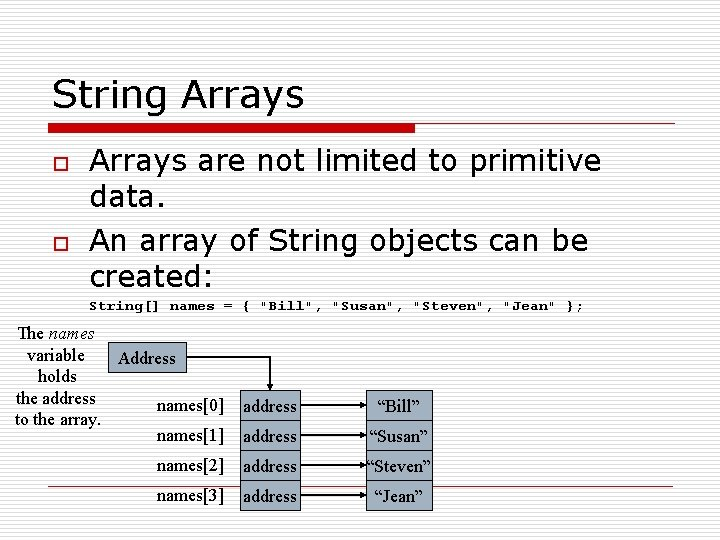String Arrays o o Arrays are not limited to primitive data. An array of String objects can be created: String[] names = { "Bill", "Susan", "Steven", "Jean" }; The names variable Address holds the address names to the array. names address “Bill” address “Susan” names address “Steven” names address “Jean”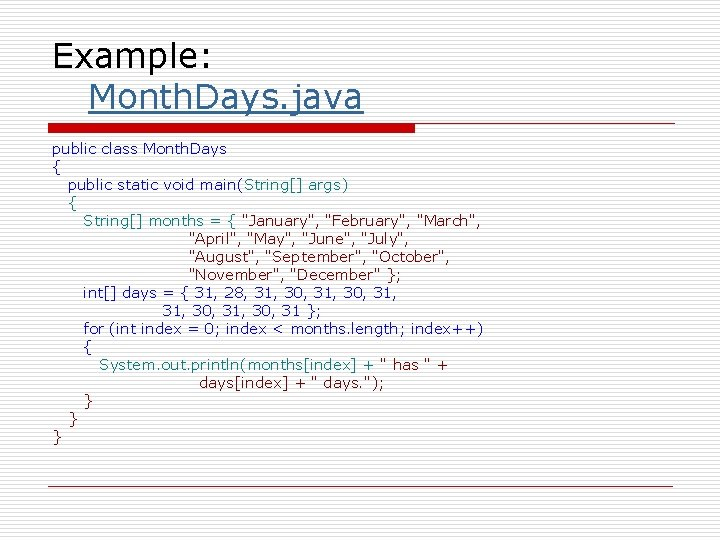Example: Month. Days. java public class Month. Days { public static void main(String[] args) { String[] months = { "January", "February", "March", "April", "May", "June", "July", "August", "September", "October", "November", "December" }; int[] days = { 31, 28, 31, 30, 31 }; for (int index = 0; index < months. length; index++) { System. out. println(months[index] + " has " + days[index] + " days. "); } } }String Arrays o If an initialization list is not provided, the new keyword must be used to create the array: String[] names = new String; The names variable holds the address to the array. Address names null names null names null names null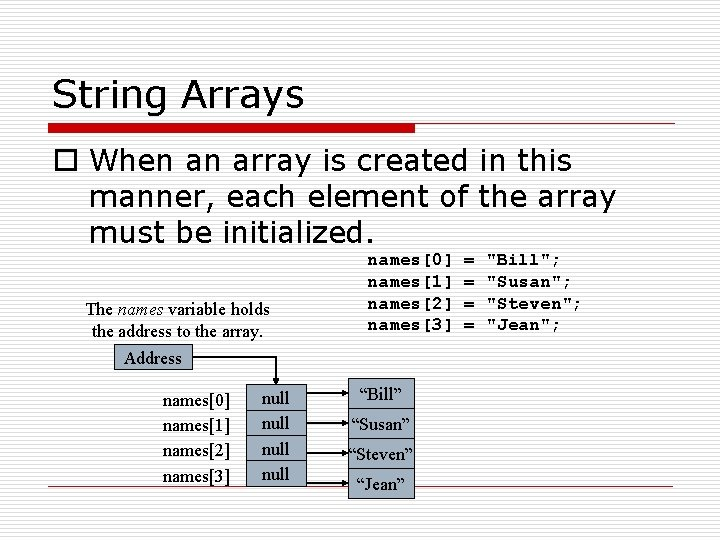String Arrays o When an array is created in this manner, each element of the array must be initialized. The names variable holds the address to the array. names names names names Address names names names names null “Bill” “Susan” “Steven” “Jean” = = "Bill"; "Susan"; "Steven"; "Jean";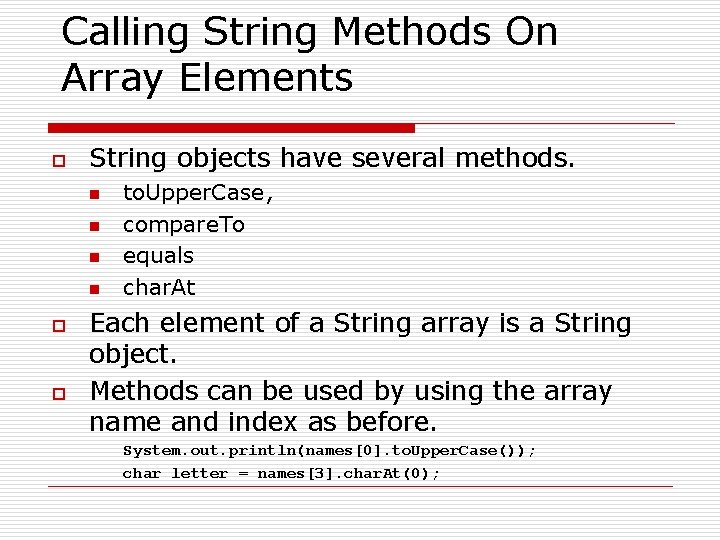Calling String Methods On Array Elements o String objects have several methods. n n o o to. Upper. Case, compare. To equals char. At Each element of a String array is a String object. Methods can be used by using the array name and index as before. System. out. println(names. to. Upper. Case()); char letter = names. char. At(0);The length Field & The length Method o o o Arrays have a final field named length. String objects have a method named length. To display the length of each string held in a String array: for (int i = 0; i < names. length; i++) System. out. println(names[i]. length()); o An array’s length is a field n o You do not write a set of parentheses after its name. A String’s length is a method n You do write the parentheses after the name of the String class’s length method.The Sequential Search Algorithm o o A search algorithm is a method of locating a specific item in a larger collection of data. The sequential search algorithm uses a loop to: n n n sequentially step through an array, compare each element with the search value, and stop when o the value is found or o the end of the array is encountered.Example: Search. Array. java public class Search. Array { public static void main(String[] args) { int[] tests = { 87, 75, 98, 100, 82 }; int results; results = sequential. Search(tests, 100); if (results == -1) { System. out. println("You did not " + "earn 100 on any test. "); } else { System. out. println("You earned 100 " + "on test " + (results + 1)); } }Example: Search. Array. java public static int sequential. Search(int[] array, int value) { int index; // Loop control variable int element; // Element the value is found at boolean found; // Flag indicating search results index = 0; element = -1; found = false; while (!found && index < array. length) { if (array[index] == value) { found = true; element = index; } index++; } return element; } }Parallel Arrays o By using the same subscript, you can build relationships between data stored in two or more arrays. String[] names = new String; String[] addresses = new String; n n n The names array stores the names of five persons The addresses array stores the addresses of the same five persons. The data for one person is stored at the same index in each array.Parallel Arrays Relationship between names and addresses array elements. names names names names names Person #1 Person #2 Person #3 Person #4 Person #5 addresses addresses addresses addresses addresses • Parallel arrays are useful when storing data of unlike types.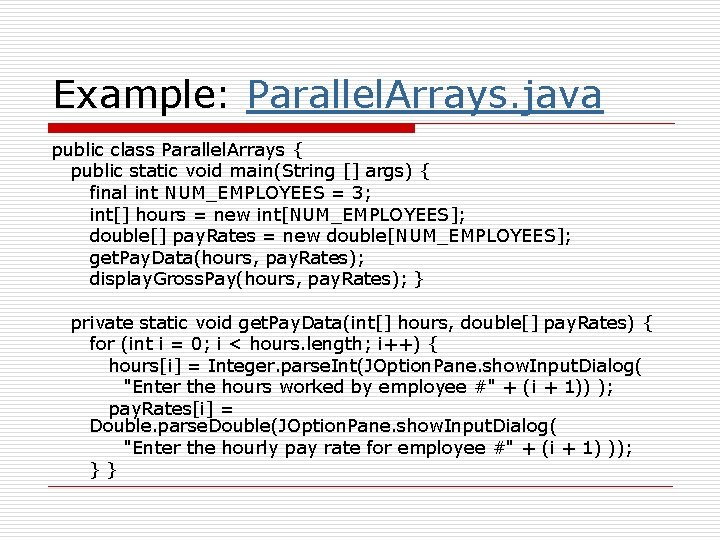Example: Parallel. Arrays. java public class Parallel. Arrays { public static void main(String [] args) { final int NUM_EMPLOYEES = 3; int[] hours = new int[NUM_EMPLOYEES]; double[] pay. Rates = new double[NUM_EMPLOYEES]; get. Pay. Data(hours, pay. Rates); display. Gross. Pay(hours, pay. Rates); } private static void get. Pay. Data(int[] hours, double[] pay. Rates) { for (int i = 0; i < hours. length; i++) { hours[i] = Integer. parse. Int(JOption. Pane. show. Input. Dialog( "Enter the hours worked by employee #" + (i + 1)) ); pay. Rates[i] = Double. parse. Double(JOption. Pane. show. Input. Dialog( "Enter the hourly pay rate for employee #" + (i + 1) )); }}Example: Parallel. Arrays. java private static void display. Gross. Pay(int [] hours, double [] pay. Rates) { double gross. Pay; // To hold gross pay Decimal. Format dollar = new Decimal. Format("#, ##0. 00"); for (int i = 0; i < hours. length; i++) { gross. Pay = hours[i] * pay. Rates[i]; System. out. println("The gross pay for " + "employee #" + (i + 1) + " is \$" + dollar. format(gross. Pay)); } } }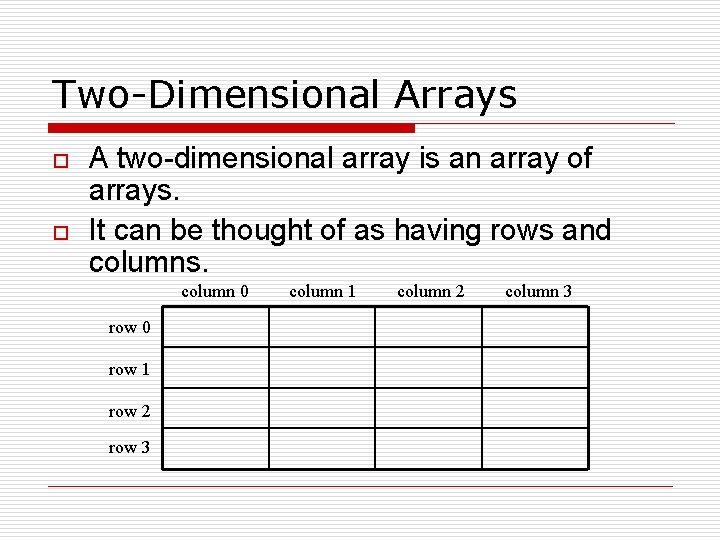Two-Dimensional Arrays o o A two-dimensional array is an array of arrays. It can be thought of as having rows and columns. column 0 row 1 row 2 row 3 column 1 column 2 column 3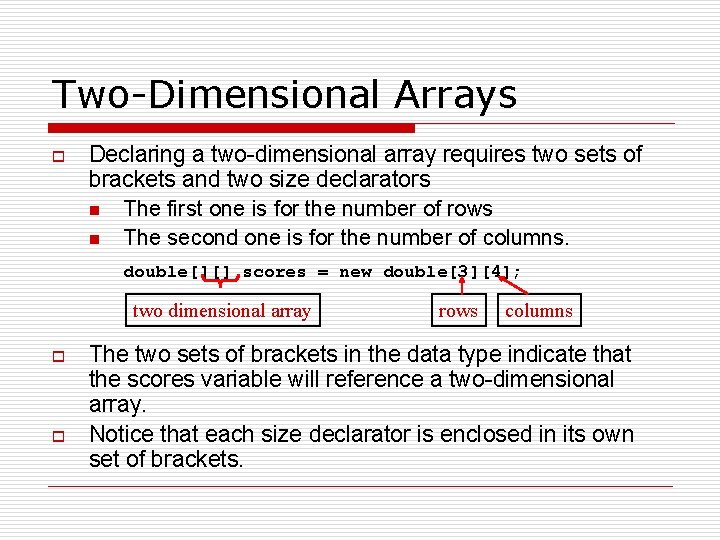Two-Dimensional Arrays o Declaring a two-dimensional array requires two sets of brackets and two size declarators n The first one is for the number of rows n The second one is for the number of columns. double[][] scores = new double; two dimensional array o o rows columns The two sets of brackets in the data type indicate that the scores variable will reference a two-dimensional array. Notice that each size declarator is enclosed in its own set of brackets.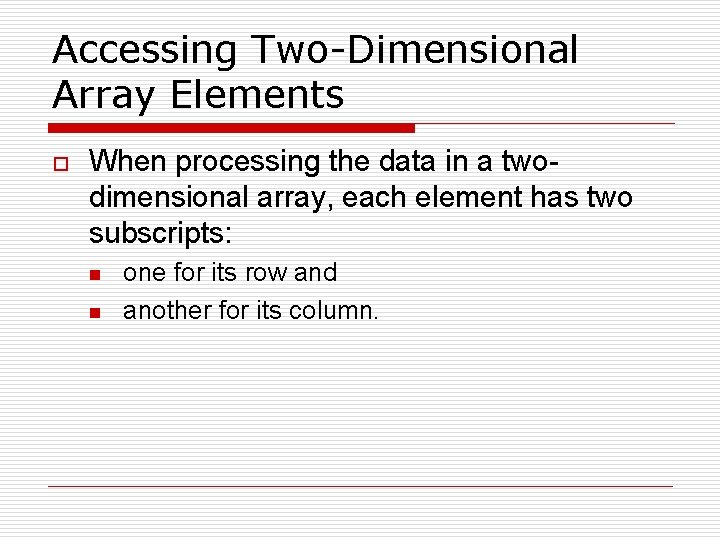Accessing Two-Dimensional Array Elements o When processing the data in a twodimensional array, each element has two subscripts: n n one for its row and another for its column.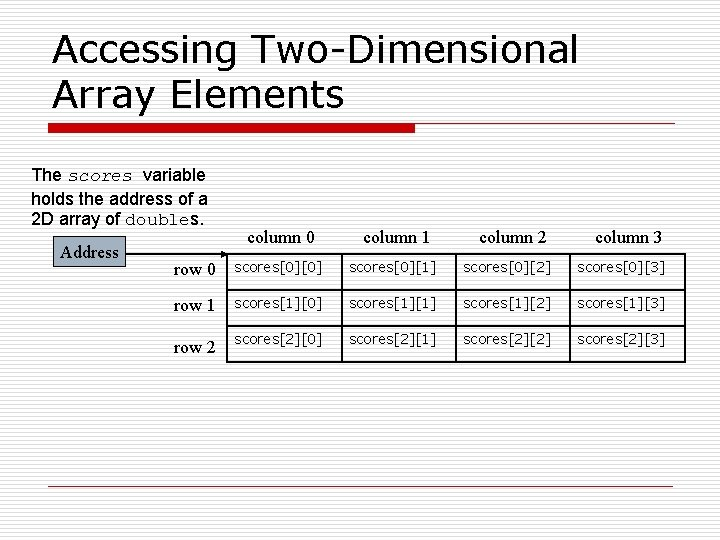Accessing Two-Dimensional Array Elements The scores variable holds the address of a 2 D array of doubles. Address column 0 column 1 column 2 column 3 row 0 scores scores scores scores row 1 scores scores scores scores scores scores scores scores row 2Accessing Two-Dimensional Array Elements The scores variable holds the address of a 2 D array of doubles. Address Accessing one of the elements in a twodimensional array requires the use of both subscripts. scores = 95; column 0 column 1 column 2 column 3 row 0 0 0 row 1 0 0 row 2 0 95 0 0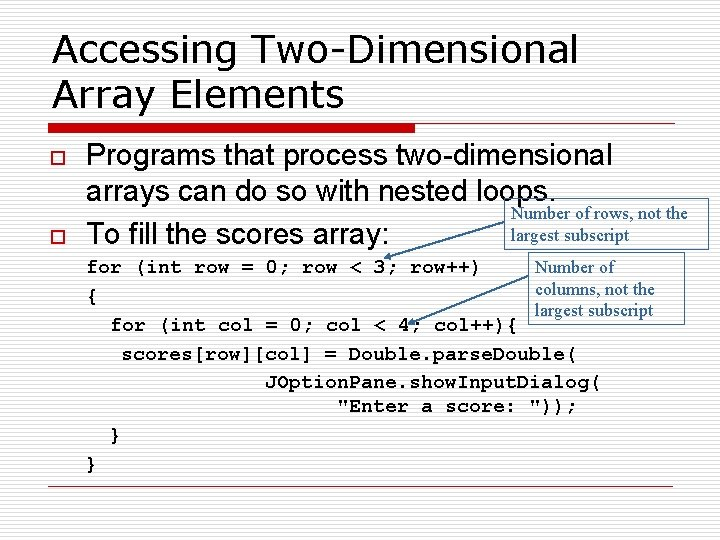Accessing Two-Dimensional Array Elements o o Programs that process two-dimensional arrays can do so with nested loops. Number of rows, not the largest subscript To fill the scores array: Number of for (int row = 0; row < 3; row++) columns, not the { largest subscript for (int col = 0; col < 4; col++){ scores[row][col] = Double. parse. Double( JOption. Pane. show. Input. Dialog( "Enter a score: ")); } }Accessing Two-Dimensional Array Elements o To print out the scores array: for (int row = 0; row < 3; row++) { for (int col = 0; col < 4; col++) { System. out. println(scores[row][col]); } }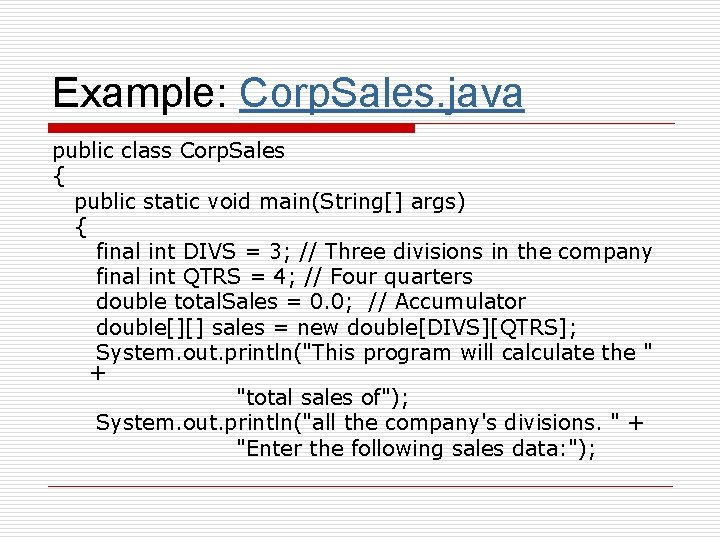Example: Corp. Sales. java public class Corp. Sales { public static void main(String[] args) { final int DIVS = 3; // Three divisions in the company final int QTRS = 4; // Four quarters double total. Sales = 0. 0; // Accumulator double[][] sales = new double[DIVS][QTRS]; System. out. println("This program will calculate the " + "total sales of"); System. out. println("all the company's divisions. " + "Enter the following sales data: ");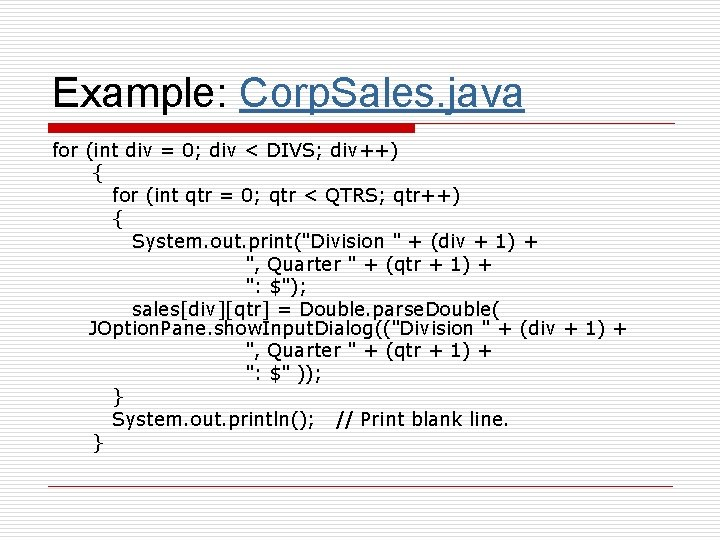Example: Corp. Sales. java for (int div = 0; div < DIVS; div++) { for (int qtr = 0; qtr < QTRS; qtr++) { System. out. print("Division " + (div + 1) + ", Quarter " + (qtr + 1) + ": \$"); sales[div][qtr] = Double. parse. Double( JOption. Pane. show. Input. Dialog(("Division " + (div + 1) + ", Quarter " + (qtr + 1) + ": \$" )); } System. out. println(); // Print blank line. }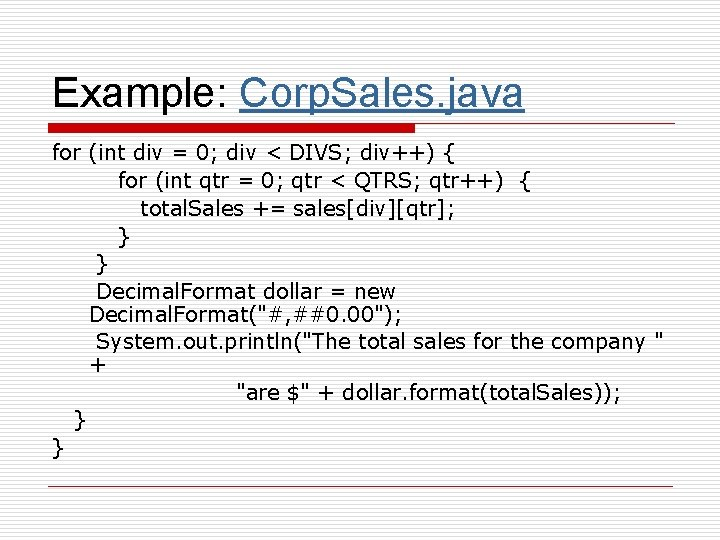Example: Corp. Sales. java for (int div = 0; div < DIVS; div++) { for (int qtr = 0; qtr < QTRS; qtr++) { total. Sales += sales[div][qtr]; } } Decimal. Format dollar = new Decimal. Format("#, ##0. 00"); System. out. println("The total sales for the company " + "are \$" + dollar. format(total. Sales)); } }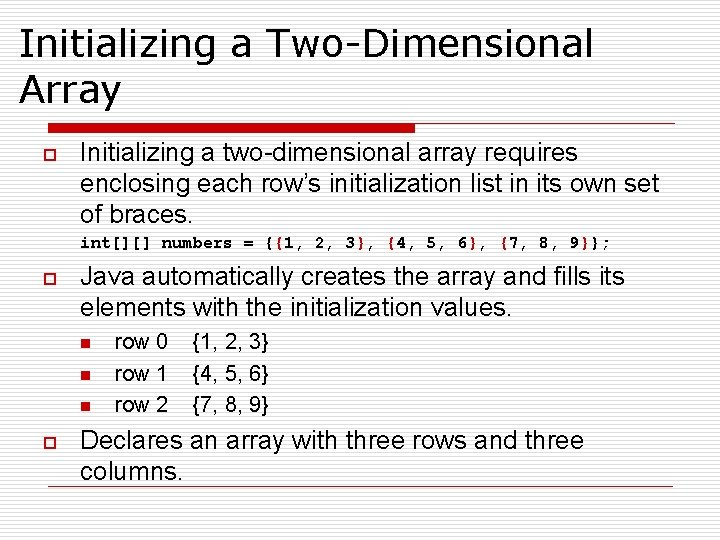Initializing a Two-Dimensional Array o Initializing a two-dimensional array requires enclosing each row’s initialization list in its own set of braces. int[][] numbers = {{1, 2, 3}, {4, 5, 6}, {7, 8, 9}}; o Java automatically creates the array and fills its elements with the initialization values. n n n o row 0 row 1 row 2 {1, 2, 3} {4, 5, 6} {7, 8, 9} Declares an array with three rows and three columns.Initializing a Two-Dimensional Array The numbers variable holds the address of a 2 D array of int values. Address int[][] numbers = {{1, 2, 3}, {4, 5, 6}, {7, 8, 9}}; produces: column 0 column 1 column 2 row 0 1 2 3 row 1 4 5 6 row 2 7 8 9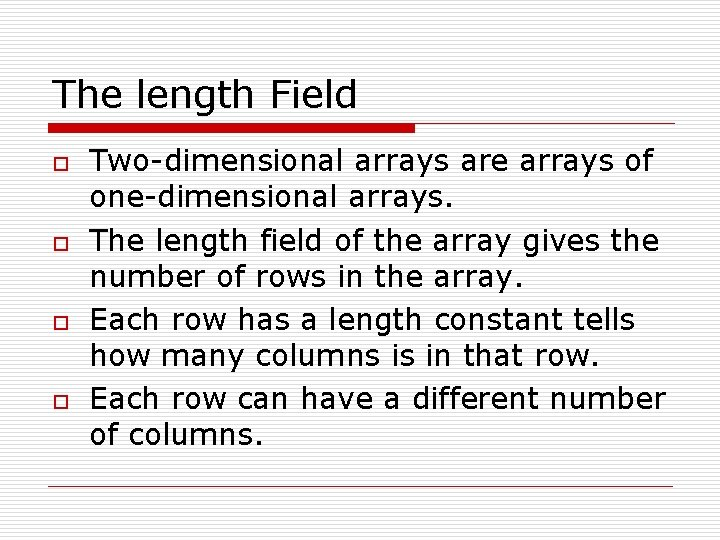The length Field o o Two-dimensional arrays are arrays of one-dimensional arrays. The length field of the array gives the number of rows in the array. Each row has a length constant tells how many columns is in that row. Each row can have a different number of columns.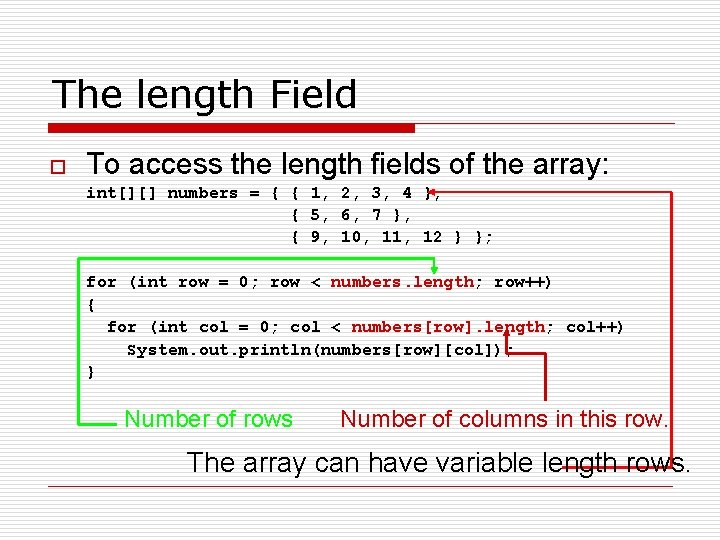The length Field o To access the length fields of the array: int[][] numbers = { { 1, 2, 3, 4 }, { 5, 6, 7 }, { 9, 10, 11, 12 } }; for (int row = 0; row < numbers. length; row++) { for (int col = 0; col < numbers[row]. length; col++) System. out. println(numbers[row][col]); } Number of rows Number of columns in this row. The array can have variable length rows.Summing The Elements of a Two-Dimensional Array int[][] numbers = { { 1, 2, 3, 4 }, {5, 6, 7, 8}, {9, 10, 11, 12} }; int total; total = 0; for (int row = 0; row < numbers. length; row++) { for (int col = 0; col < numbers[row]. length; col++) total += numbers[row][col]; } System. out. println("The total is " + total);Example: Lengths. java public class Lengths { public static void main(String[] args) { int[][] numbers = { { 1, 2, 3, 4 }, { 5, 6, 7, 8 }, { 9, 10, 11, 12 } }; System. out. println("The number of " + "rows is " + numbers. length); for (int index = 0; index < numbers. length; index++) { System. out. println("The number of " + "columns in row " + index + " is " + numbers[index]. length); } } }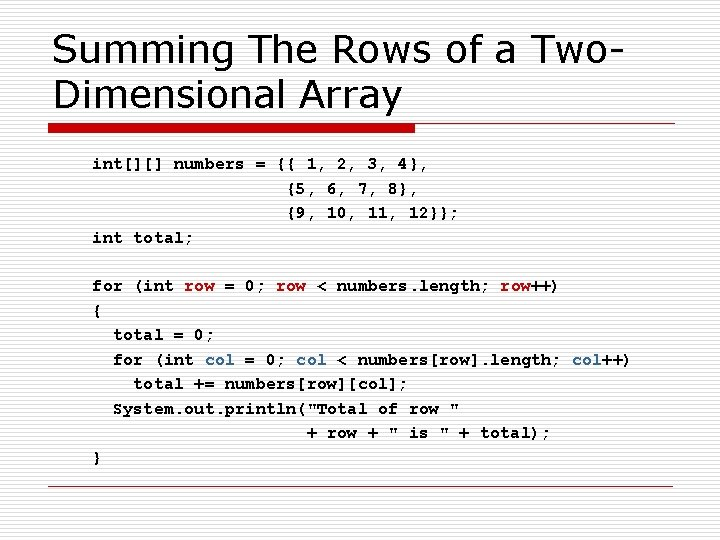Summing The Rows of a Two. Dimensional Array int[][] numbers = {{ 1, 2, 3, 4}, {5, 6, 7, 8}, {9, 10, 11, 12}}; int total; for (int row = 0; row < numbers. length; row++) { total = 0; for (int col = 0; col < numbers[row]. length; col++) total += numbers[row][col]; System. out. println("Total of row " + row + " is " + total); }Summing The Columns of a Two-Dimensional Array int[][] numbers = {{1, 2, 3, 4}, {5, 6, 7, 8}, {9, 10, 11, 12}}; int total; for (int col = 0; col < numbers. length; col++) { total = 0; for (int row = 0; row < numbers. length; row++) total += numbers[row][col]; System. out. println("Total of column " + col + " is " + total); }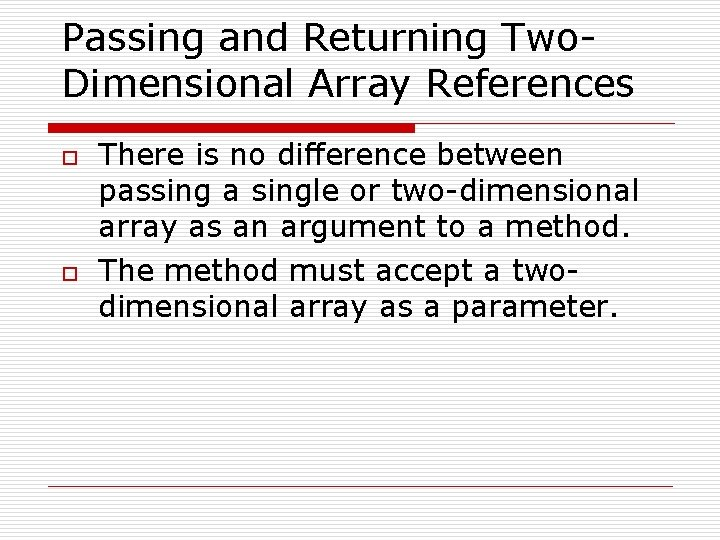Passing and Returning Two. Dimensional Array References o o There is no difference between passing a single or two-dimensional array as an argument to a method. The method must accept a twodimensional array as a parameter.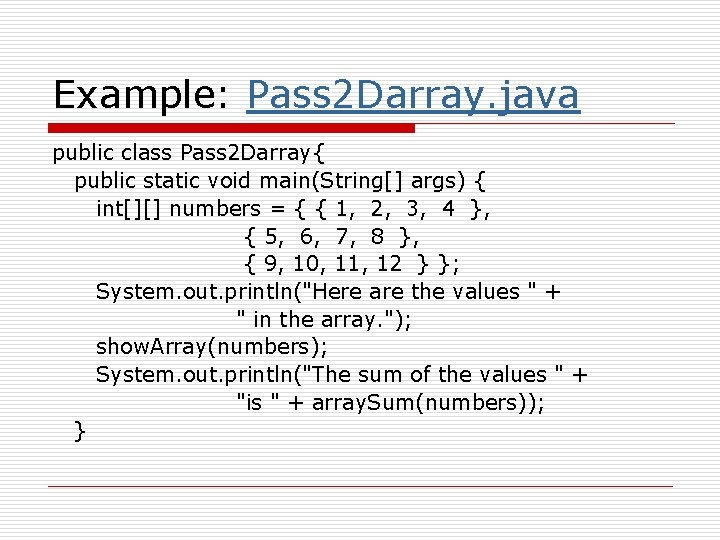Example: Pass 2 Darray. java public class Pass 2 Darray{ public static void main(String[] args) { int[][] numbers = { { 1, 2, 3, 4 }, { 5, 6, 7, 8 }, { 9, 10, 11, 12 } }; System. out. println("Here are the values " + " in the array. "); show. Array(numbers); System. out. println("The sum of the values " + "is " + array. Sum(numbers)); }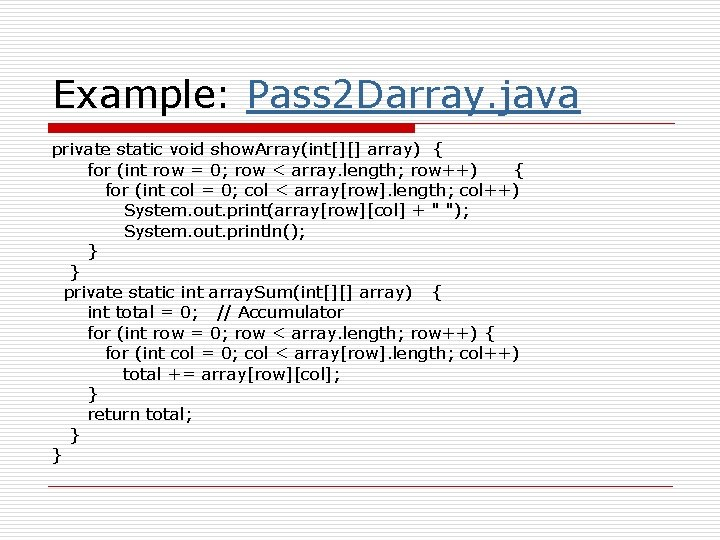Example: Pass 2 Darray. java private static void show. Array(int[][] array) { for (int row = 0; row < array. length; row++) { for (int col = 0; col < array[row]. length; col++) System. out. print(array[row][col] + " "); System. out. println(); } } private static int array. Sum(int[][] array) { int total = 0; // Accumulator for (int row = 0; row < array. length; row++) { for (int col = 0; col < array[row]. length; col++) total += array[row][col]; } return total; } }Ragged Arrays o o When the rows of a two-dimensional array are of different lengths, the array is known as a ragged array. You can create a ragged array by creating a two-dimensional array with a specific number of rows, but no columns. int [][] ragged = new int []; o Then create the individual rows. ragged ragged ragged ragged = = new new int int ; ; ; ;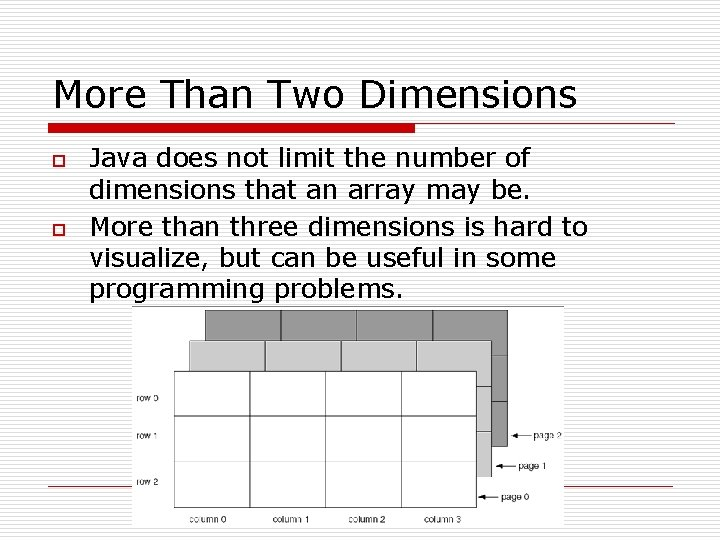More Than Two Dimensions o o Java does not limit the number of dimensions that an array may be. More than three dimensions is hard to visualize, but can be useful in some programming problems.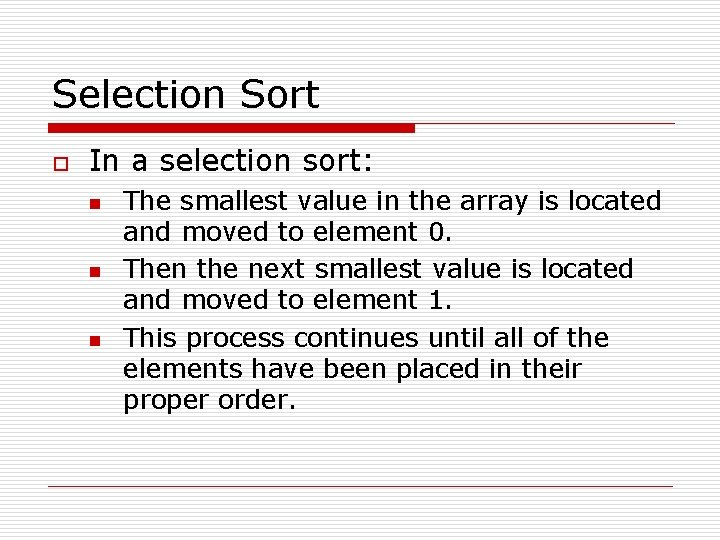Selection Sort o In a selection sort: n n n The smallest value in the array is located and moved to element 0. Then the next smallest value is located and moved to element 1. This process continues until all of the elements have been placed in their proper order.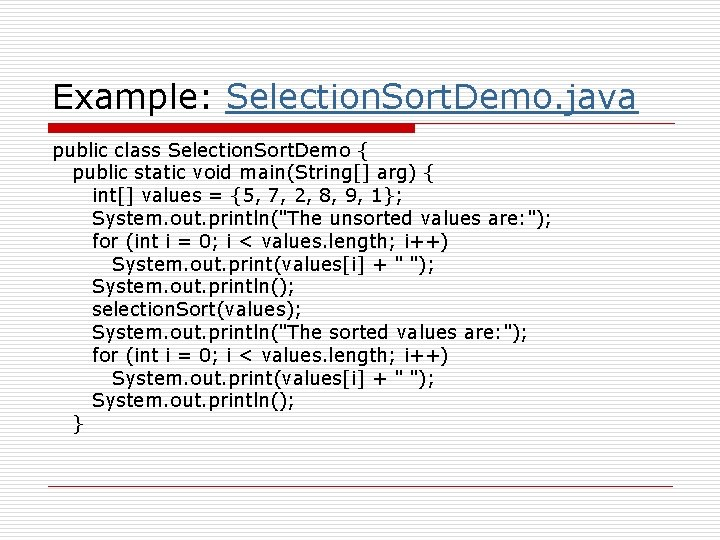Example: Selection. Sort. Demo. java public class Selection. Sort. Demo { public static void main(String[] arg) { int[] values = {5, 7, 2, 8, 9, 1}; System. out. println("The unsorted values are: "); for (int i = 0; i < values. length; i++) System. out. print(values[i] + " "); System. out. println(); selection. Sort(values); System. out. println("The sorted values are: "); for (int i = 0; i < values. length; i++) System. out. print(values[i] + " "); System. out. println(); }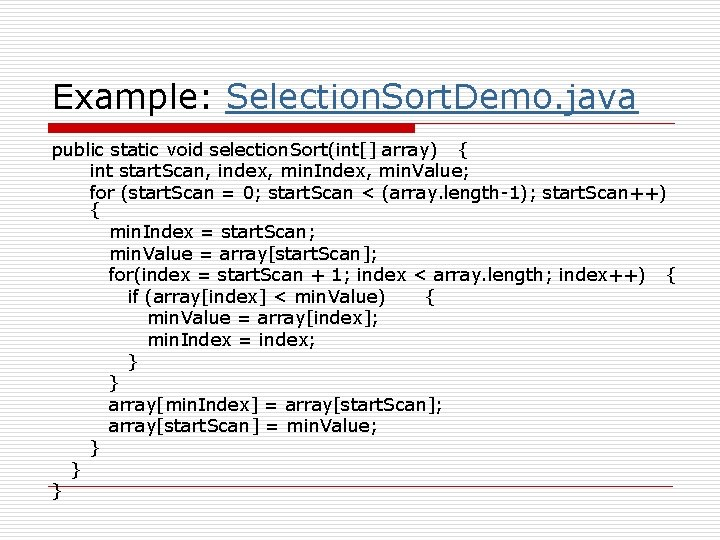Example: Selection. Sort. Demo. java public static void selection. Sort(int[] array) { int start. Scan, index, min. Index, min. Value; for (start. Scan = 0; start. Scan < (array. length-1); start. Scan++) { min. Index = start. Scan; min. Value = array[start. Scan]; for(index = start. Scan + 1; index < array. length; index++) { if (array[index] < min. Value) { min. Value = array[index]; min. Index = index; } } array[min. Index] = array[start. Scan]; array[start. Scan] = min. Value; } } }Binary Search o A binary search: n n n n requires an array sorted in ascending order. starts with the element in the middle of the array. If that element is the desired value, the search is over. Otherwise, the value in the middle element is either greater or less than the desired value If it is greater than the desired value, search in the first half of the array. Otherwise, search the last half of the array. Repeat as needed while adjusting start and end points of the search.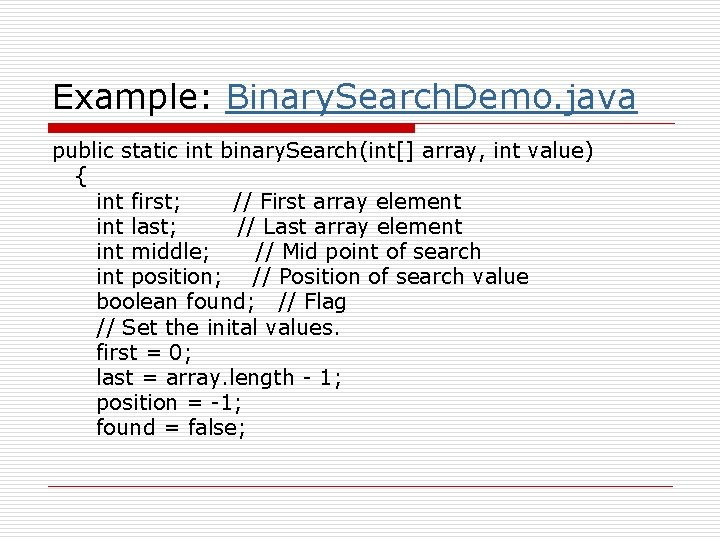Example: Binary. Search. Demo. java public static int binary. Search(int[] array, int value) { int first; // First array element int last; // Last array element int middle; // Mid point of search int position; // Position of search value boolean found; // Flag // Set the inital values. first = 0; last = array. length - 1; position = -1; found = false;Binary. Search. Demo. java while (!found && first <= last) { // Calculate mid point middle = (first + last) / 2; // If value is found at midpoint. . . if (array[middle] == value) { found = true; position = middle; } // else if value is in lower half. . . else if (array[middle] > value) last = middle - 1; // else if value is in upper half. . else first = middle + 1; } } return position;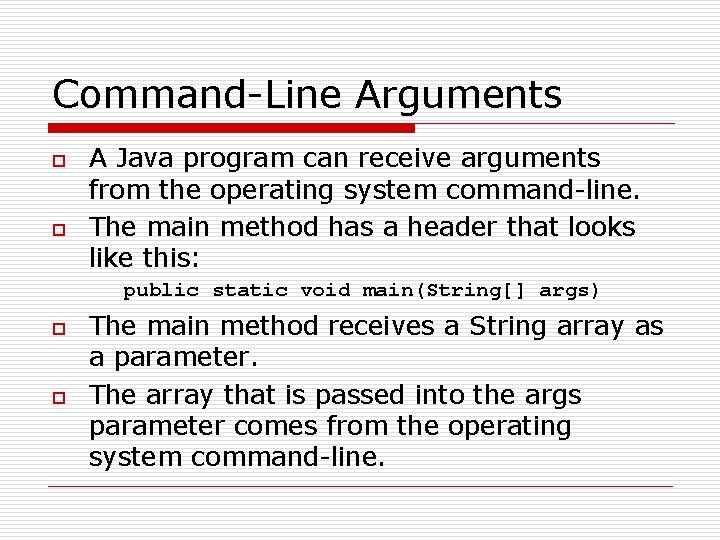Command-Line Arguments o o A Java program can receive arguments from the operating system command-line. The main method has a header that looks like this: public static void main(String[] args) o o The main method receives a String array as a parameter. The array that is passed into the args parameter comes from the operating system command-line.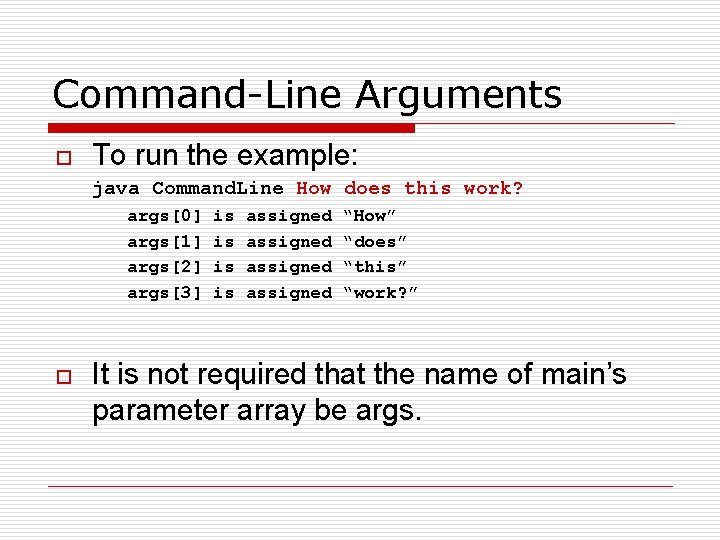Command-Line Arguments o To run the example: java Command. Line How does this work? args args args args o is is assigned “How” “does” “this” “work? ” It is not required that the name of main’s parameter array be args.Examples: Command. Line. java public class Command. Line { public static void main(String[] args) { for (int index = 0; index < args. length; index++) System. out. println(args[index]); } }